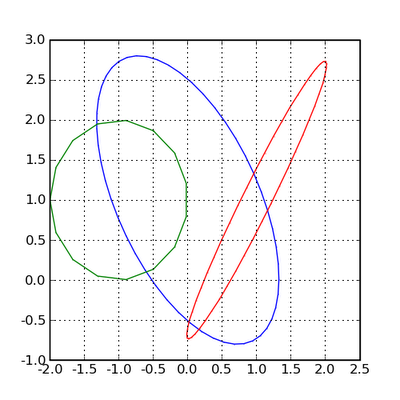Wednesday, November 26, 2008

Python: draw an ellipse

The following Python script draws an ellipse.
`from numpy import linspacefrom scipy import pi,sin,cosdef ellipse(ra,rb,ang,x0,y0,Nb=50):'''ra - major axis length rb - minor axis length ang - angle x0,y0 - position of centre of ellipse Nb - No. of points that make an ellipse based on matlab code ellipse.m written by D.G. Long, Brigham Young University, based on the CIRCLES.m original written by Peter Blattner, Institute of Microtechnology, University of Neuchatel, Switzerland, blattner@imt.unine.ch'''xpos,ypos=x0,y0radm,radn=ra,rban=angco,si=cos(an),sin(an)the=linspace(0,2*pi,Nb)X=radm*cos(the)*co-si*radn*sin(the)+xposY=radm*cos(the)*si+co*radn*sin(the)+yposreturn X,Ydef test():import pylab as pfig = p.figure(figsize=(5,5))p.axis([-3,3,-3,3])#eg 1X,Y=ellipse(2,1,pi*2.0/3.0,0,1)p.plot(X,Y,"b.-",ms=1) # blue ellipse#eg 2X,Y=ellipse(2,0.2,pi/3.0,1,1)p.plot(X,Y,"r.-",ms=1) # red ellipse#eg 3X,Y=ellipse(1,1,pi/3.0,-1,1,Nb=16)p.plot(X,Y,"g.-",ms=1) # green ellipsep.grid(True)p.show()if __name__ == '__main__':test()`Note

The script is in ellipse.py.

1 comment:

1.Anonymous1:36 PM

Useful, thank you.